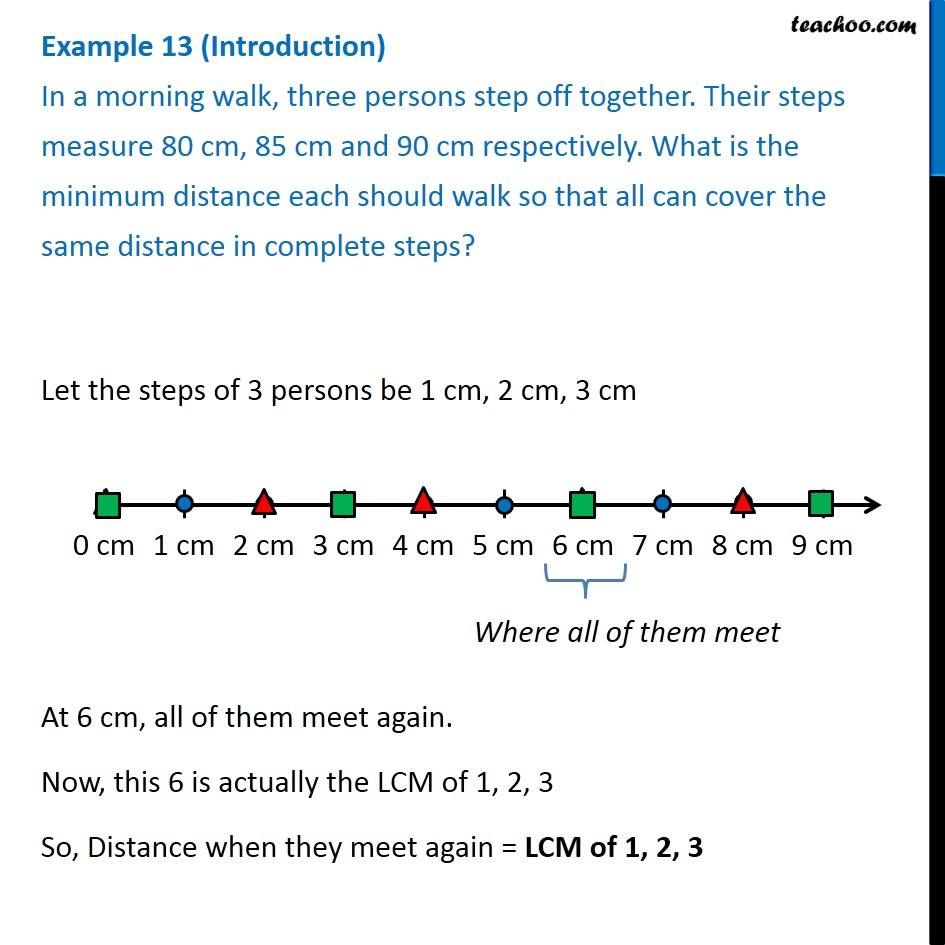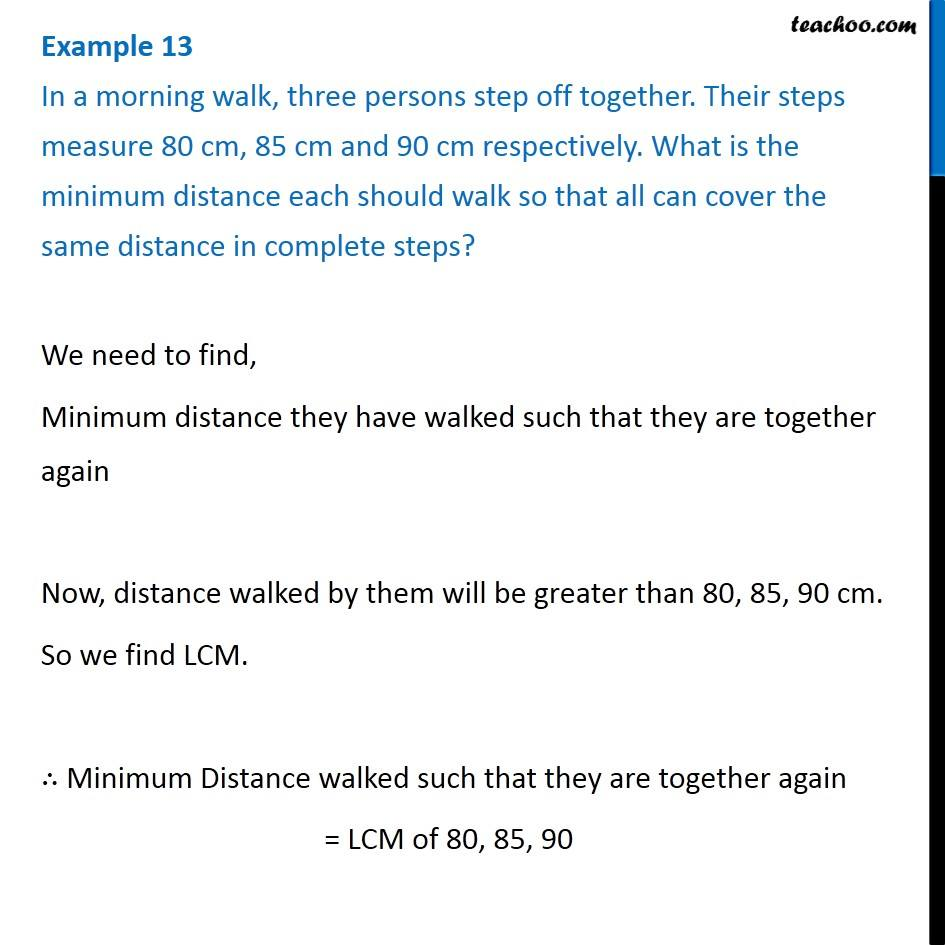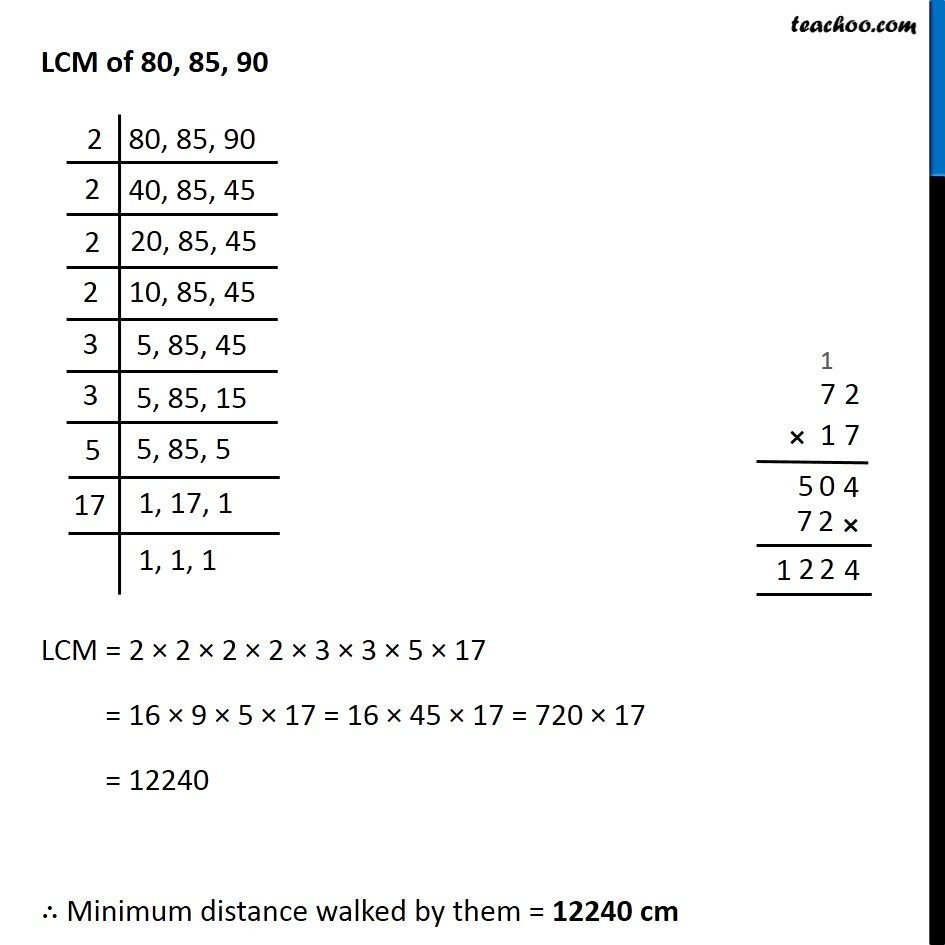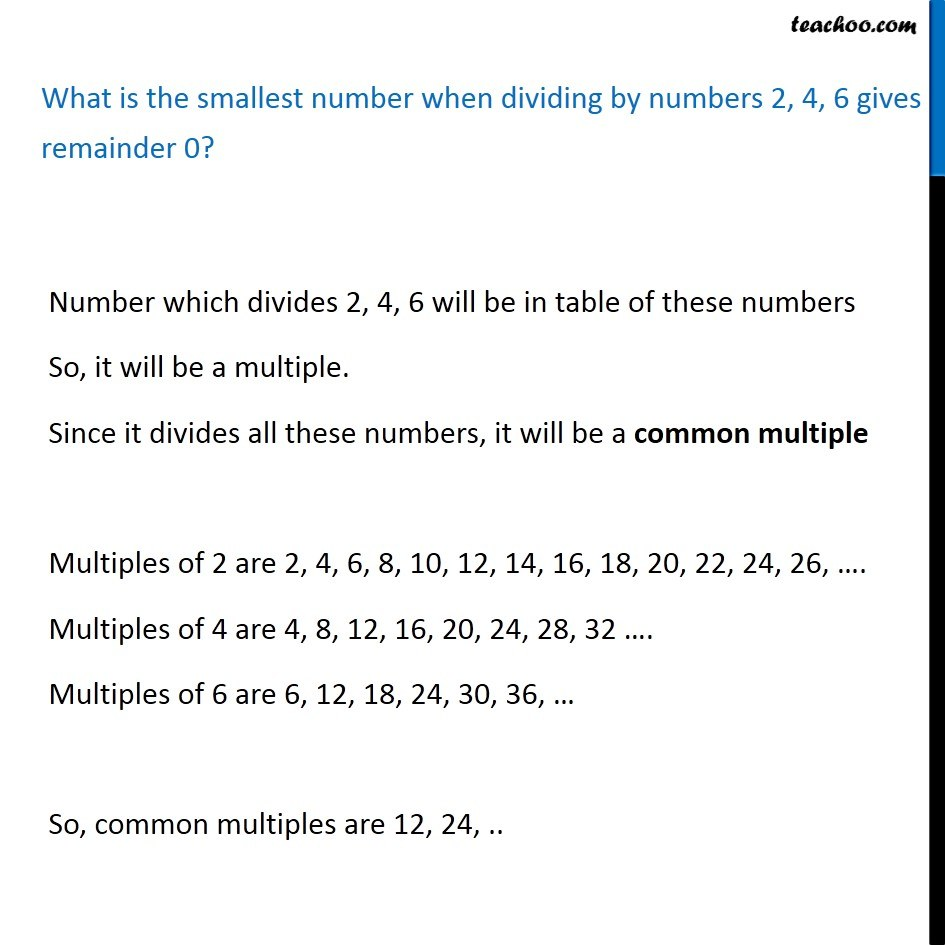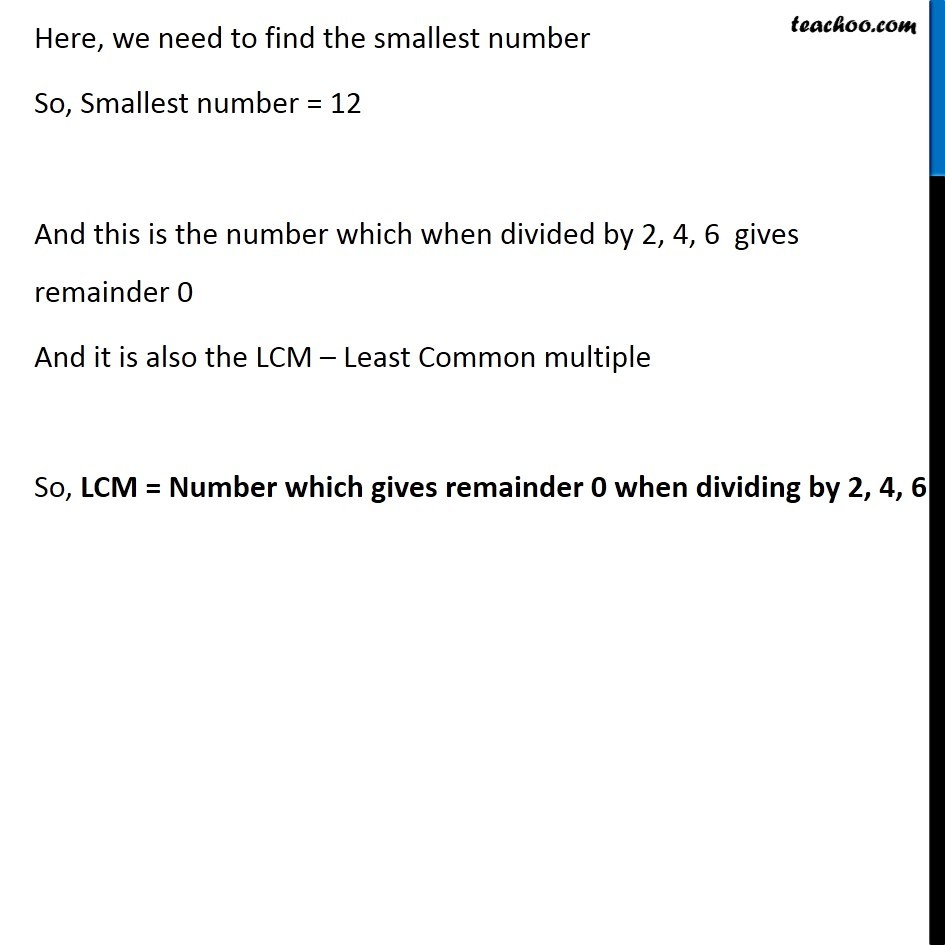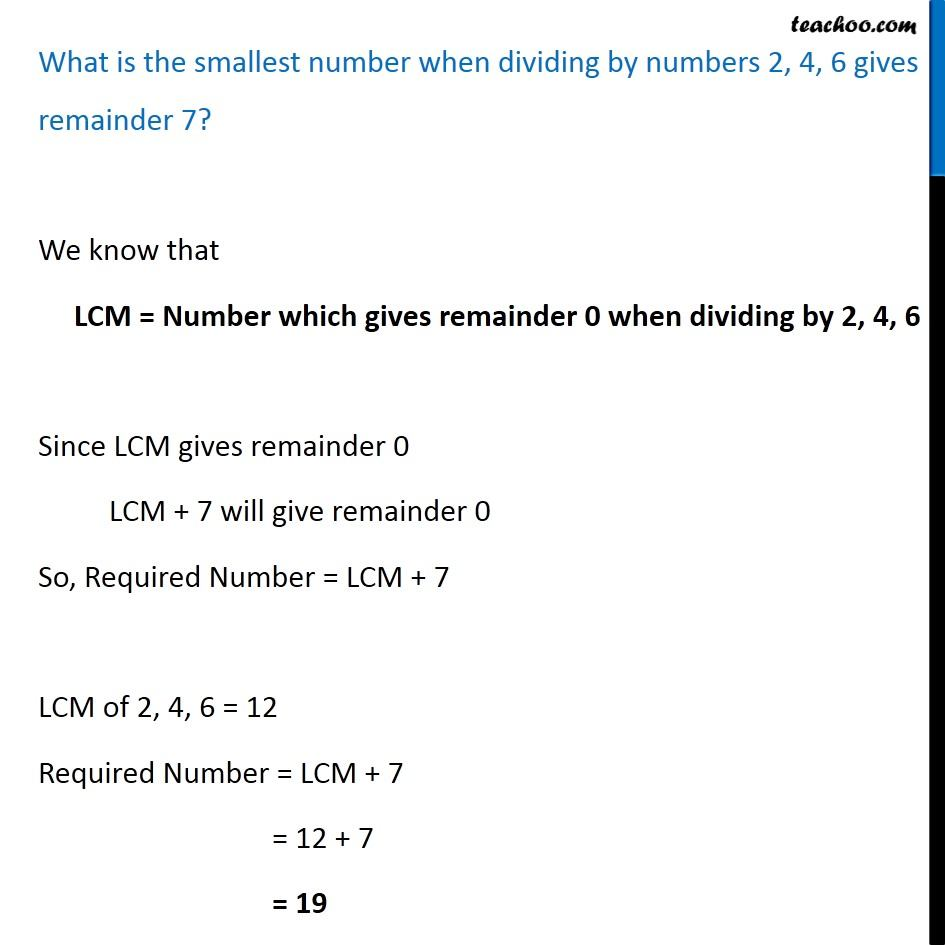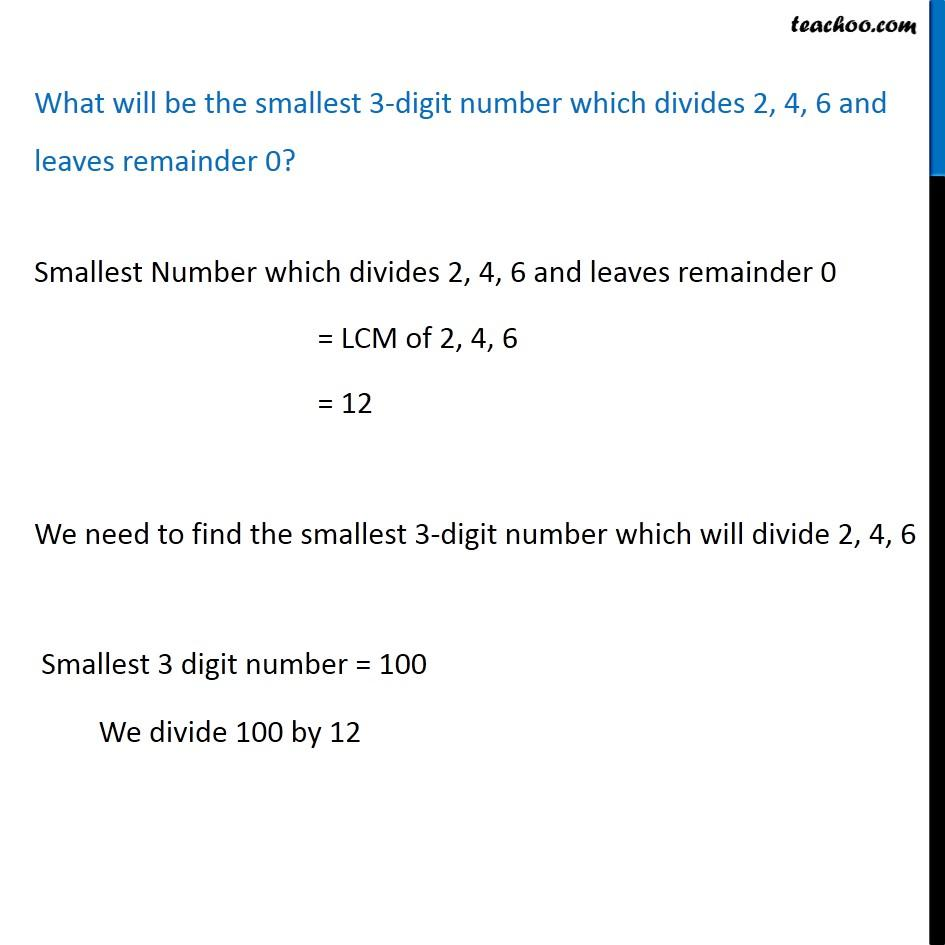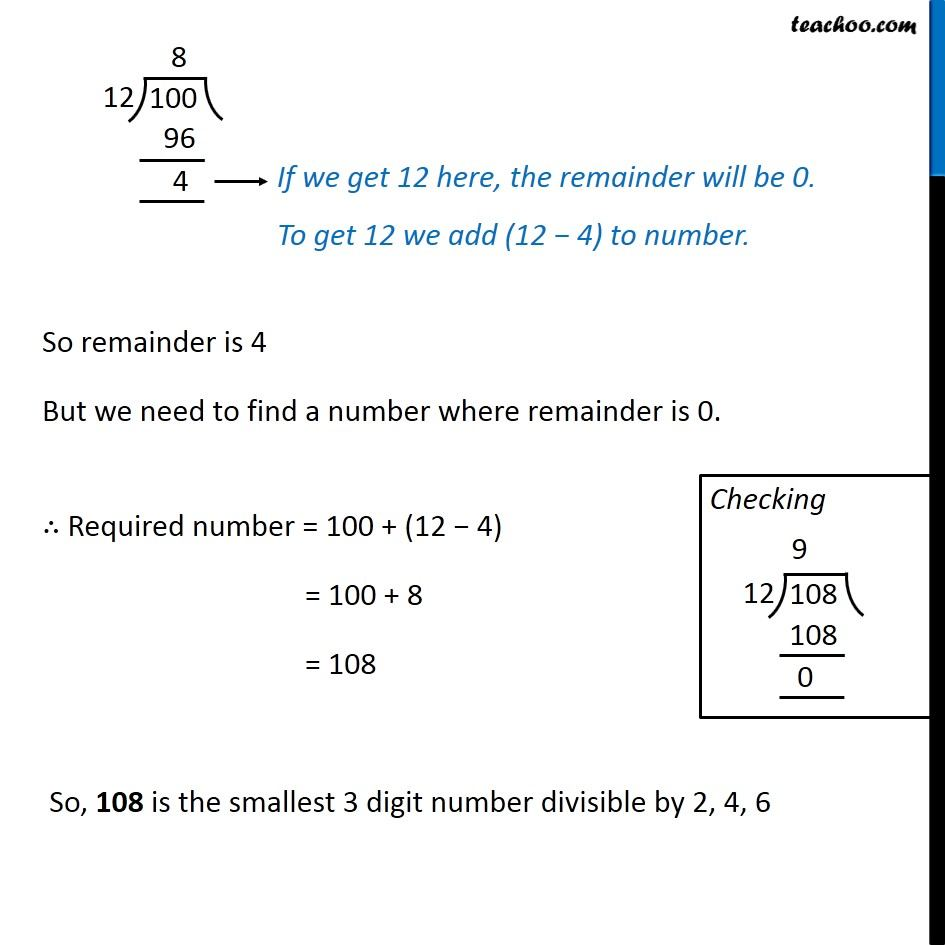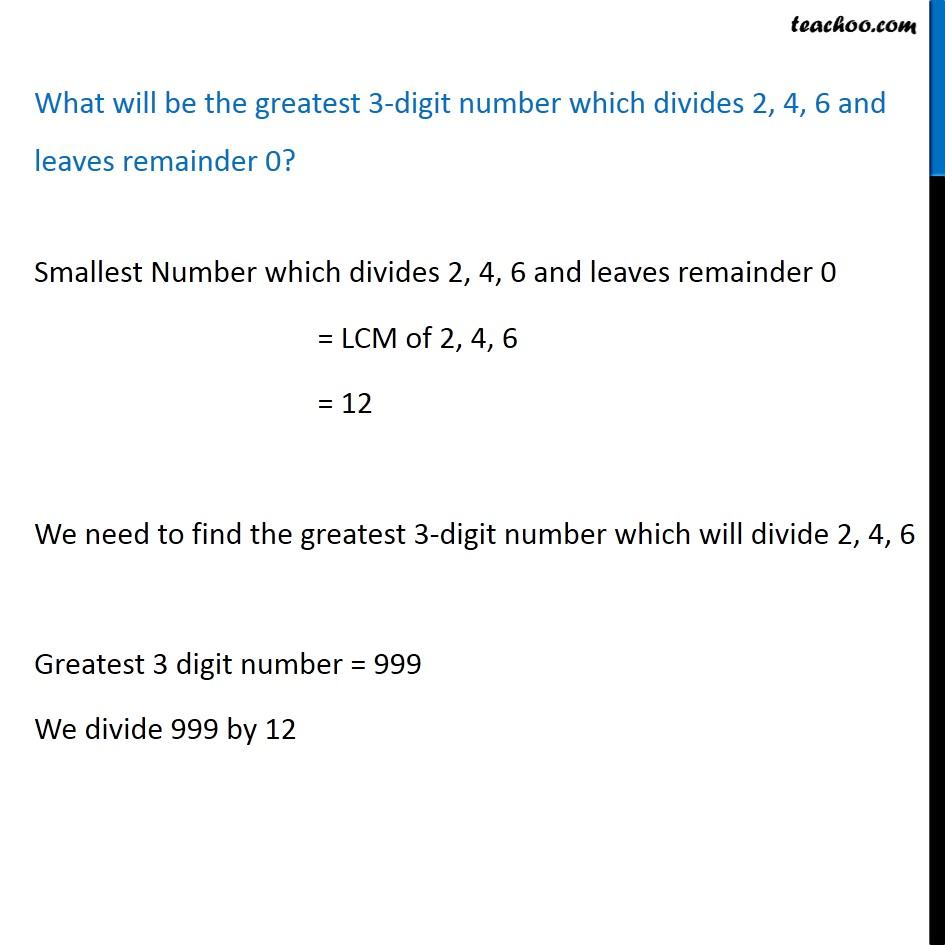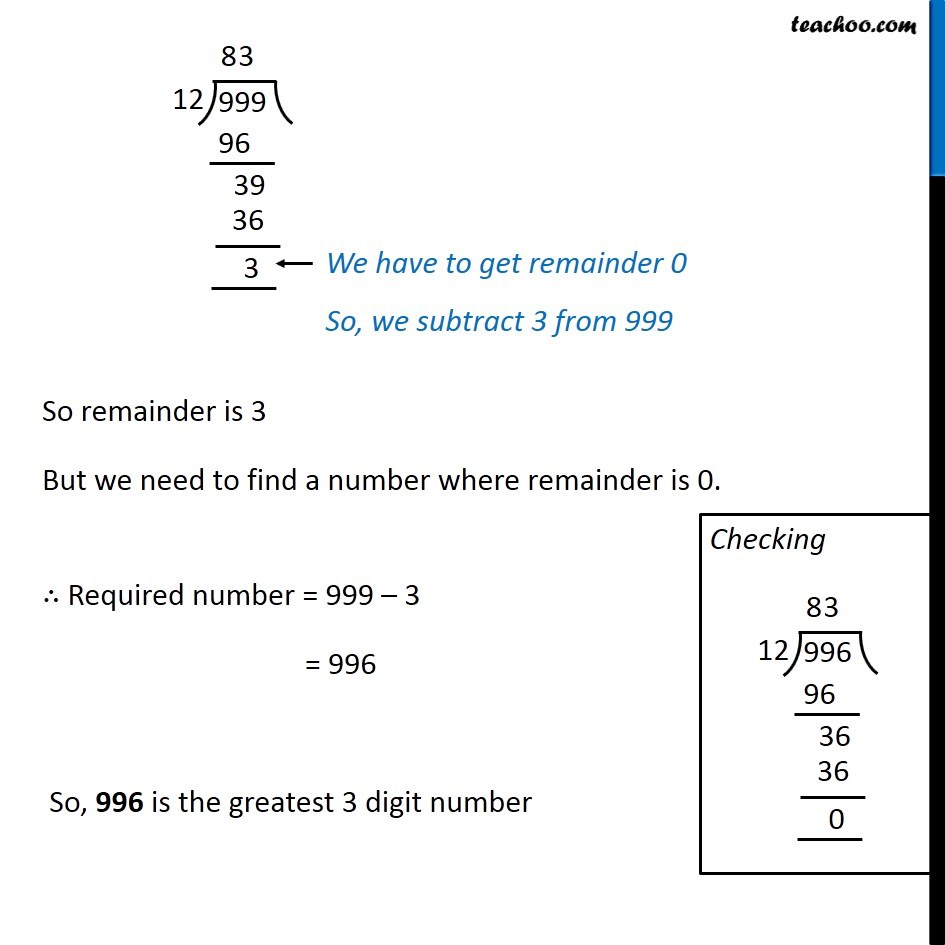1. Chapter 3 Class 6 Playing with Numbers
2. Serial order wise
3. Examples

Transcript

Example 13 (Introduction) In a morning walk, three persons step off together. Their steps measure 80 cm, 85 cm and 90 cm respectively. What is the minimum distance each should walk so that all can cover the same distance in complete steps? Let the steps of 3 persons be 1 cm, 2 cm, 3 cm At 6 cm, all of them meet again. Now, this 6 is actually the LCM of 1, 2, 3 So, Distance when they meet again = LCM of 1, 2, 3 Example 13 In a morning walk, three persons step off together. Their steps measure 80 cm, 85 cm and 90 cm respectively. What is the minimum distance each should walk so that all can cover the same distance in complete steps? Steps of 3 persons are 80 cm, 85 cm and 90 cm We need to find, Minimum distance they have walked such that they are together again Now, distance walked by them will be greater than 80, 85, 90 cm. So we find LCM. LCM of 80, 85, 90 LCM = 2 2 2 2 5 3 3 17 = 16 5 9 17 = 80 9 17 = 720 17 = 12240 Minimum distance walked by them = 12240 cm